How to Learn in 24 Hours?The Rapid Learning Movie

 Need Help? M-F: 9am-5pm(PST): Toll-Free: (877) RAPID-10 US Direct: (714) 692-2900 Int'l: 001-714-692-2900 24/7 Online Technical Support: The Rapid Support Center Secure Online Order:Need Proof? Testimonials by Our Users

 Rapid Learning Courses: MCAT in 24 Hours (2015-16) USMLE in 24 Hours (Boards) Chemistry in 24 Hours Biology in 24 Hours Physics in 24 Hours Mathematics in 24 Hours Psychology in 24 Hours SAT in 24 Hours ACT in 24 Hours AP in 24 Hours CLEP in 24 Hours DAT in 24 Hours (Dental) OAT in 24 Hours (Optometry) PCAT in 24 Hours (Pharmacy) Nursing Entrance Exams Certification in 24 Hours eBook - Survival Kits Audiobooks (MP3)

 Tell-A-Friend: Have friends taking science and math courses too? Tell them about our rapid learning system.Home »  Mathematics »  High School Algebra 2

Linear Equations

 Topic Review on "Title": Equations:  Equations are statements where two quantities have the same value. Golden Rule: Whenever the same arithmetic operations are performed on both sides the equation will remain true. Domain:  The domain is the set of all possible values that the variables can have. Solution Set: The solution set is the collection of all roots of an equation. Equivalent Equations: Equivalent equations are equations that have the same solution set. Proportions: Proportions are equations that state that two fractions are equal. Identity: An identity is an equation with one variable that has no solution. Inequality Signs: The inequality signs are read from left to right and are defined as:a is less than b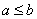a is less than or equal to b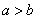a is greater than or equal to b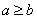a is greater than or equal to b

Rapid Study Kit for "Title":
 Flash Movie Flash Game Flash Card Core Concept Tutorial Problem Solving Drill Review Cheat Sheet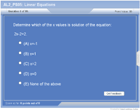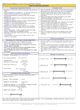"Title" Tutorial Summary : This tutorial describes what linear equations are and how they are used in application problems. Arithmetic multiplication and division operations techniques are used to show the importance of solving linear equations for unknown variables. Linear operations can be solved through the use of inverse operations. Examples are presented to show proportions and linear inequalities. Solving techniques are used to show the relationship between linear equations and linear inequalities. Finally, multiple inequalities are solved through the use of an example.

 Tutorial Features: Specific Tutorial Features: • Step by step examples showing solving linear equations, solving proportions and linear inequalities are shown in this tutorial. • Examples displaying how linear inequalities are solved and how the solutions are represented using interval notation are mentioned in this tutorial. Series Features: • Concept map showing inter-connections of new concepts in this tutorial and those previously introduced. • Definition slides introduce terms as they are needed. • Visual representation of concepts • Animated examples—worked out step by step • A concise summary is given at the conclusion of the tutorial.

 "Title" Topic List: Definition of linear equationsGolden RuleHow an equation is solvedLinear inequalities and their definition

See all 24 lessons in High School Algebra 2, including concept tutorials, problem drills and cheat sheets:
Teach Yourself High School Algebra 2 Visually in 24 Hours